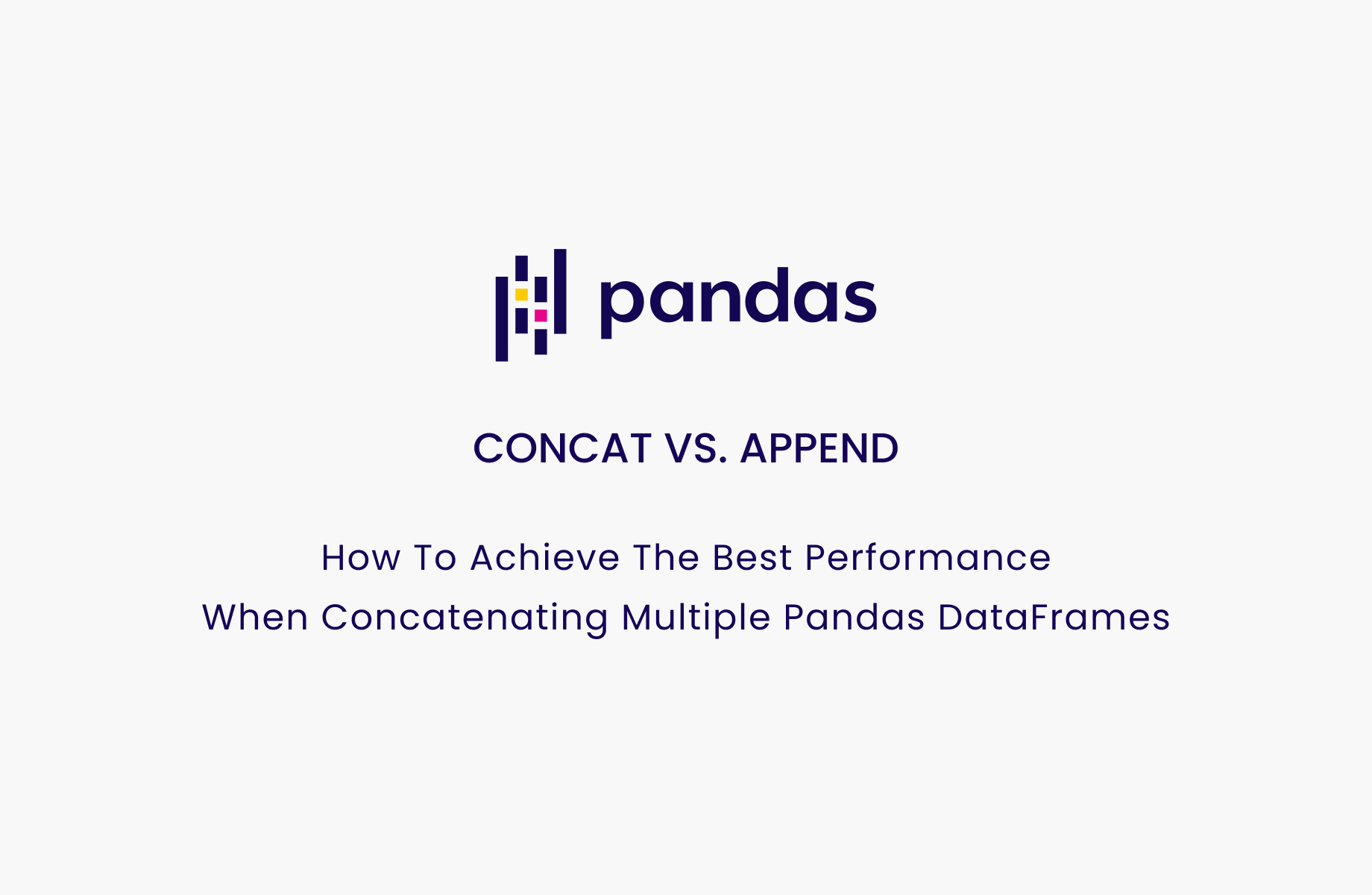# Concat vs append. How to achieve the best performance when concatenating multiple pandas DataFrames.

November 11, 2021Lucas Guillermou

Whether it is to concatenate several datasets from different csv files or to merge sets of aggregated data from different google analytics accounts, combining data from various sources is critical to drawing the right conclusions and extracting optimal value from data analytics.

When using pandas, data scientists often have to concatenate multiple pandas DataFrame; either vertically (adding lines) or horizontally (adding columns).

• Two main methods to vertically concatenate multiple pandas dataframes.
• DataFrame.append vs Pandas.concat. Which one to choose?

### I. TWO MAIN WAYS TO VERTICALLY CONCATENATE DATA FRAMES

#### DataFrame.append

This method allows you to add another dataframe to an existing one. While columns with matching names are concatenated together, columns with different labels are filled with NA.

-- CODE language-python --
>>> df1
ints  bools
0     0   True
1     1  False
2     2   True

>>> df2
ints  floats
0     3     1.5
1     4     2.5
2     5     3.5

>>> df1.append(df2)
ints  bools  floats
0     0   True     NaN
1     1  False     NaN
2     2   True     NaN
0     3    NaN     1.5
1     4    NaN     2.5
2     5    NaN     3.5

In addition to this, DataFrame.append provides you with other flexibilities such as resetting the resulting index, sorting the resulting data or raising an error when the resulting index includes duplicate records.

#### Pandas.concat

While you can concat dataframes both vertically (axis=0) and horizontally (axis=1) by using the Pandas.concat function, its main advantage lies in enabling you to vertically concatenate more than two DataFrame and/or Series at once. Unlike DataFrame.append, Pandas.concat is not a method but a function that takes a list of objects as input.

On the other hand, columns with different labels are filled with NA values as for DataFrame.append.

-- CODE language-python --
>>> df3
bools  floats
0  False     4.5
1   True     5.5
2  False     6.5

>>> pd.concat([df1, df2, df3])
ints  bools  floats
0   0.0   True     NaN
1   1.0  False     NaN
2   2.0   True     NaN
0   3.0    NaN     1.5
1   4.0    NaN     2.5
2   5.0    NaN     3.5
0   NaN  False     4.5
1   NaN   True     5.5
2   NaN  False     6.5

Furthermore, Pandas.concat enables you to modify the results by using specific parameters.

As for the horizontal concatenation, using Pandas.concat is not much different than the DataFrame.join method.

### II. SHOULD YOU CHOOSE DATAFRAME.APPEND OR PANDAS.CONCAT

#### Time is of the essence; which one is faster?

If you need to concatenate many dataframes and speed is critical, Pandas.concat is the smart choice compared to DataFrame.append. Let's figure out the difference in performance with the following code snippet:

-- CODE language-python --
df = pd.DataFrame(range(1_000_000))
dfs = [df] * 100

%%time
df_result = dfs
for df in dfs[1:]:
df_result = df_result.append(df)
CPU times: user 7.92 s, sys: 6.28 s, total: 14.2 s
Wall time: 14.2 s

%%time
df_result = pd.concat(dfs)
CPU times: user 157 ms, sys: 134 ms, total: 291 ms
Wall time: 289 ms

In this benchmark, concatenating multiple dataframes by using the Pandas.concat function is 50 times faster than using the DataFrame.append version. With multiple append , a new DataFrame is created at each iteration, and the underlying data is copied each time. These extra memory copies are very costly and cause performance issues. Let's see the memory state when concatenating 5 dataframes to have a better understanding of what happens.

We can see multiple append creates many extra copies. The space complexity of chaining append is quadratic (O(n^2)) regarding the number of inputs to concatenate. While this extra memory may be freed by the Python garbage collector, creating these blocks takes up extra time.

#### Fitness for concatenating more than two DataFrames?

In most data science projects, you will likely need to concatenate more than two objects.

If this is the case, the use of Pandas.concat instead of DataFrame.append is recommended for performance reasons.

If you are concatenating only two objects, you may prefer using DataFrame.append as it is more visible that you are concatenating vertically. In contrast, axis parameters of Pandas.concat may be visually ambiguous related to whether you are actually adding lines or columns.

### SOMETIMES ONE LINE OF CODE WORKS WONDERS

#### Concatenate different files faster without MemoryError using Terality

At Terality we are building the fully hosted, scalable and lightning fast pandas data processing engine. By only changing the import line, we allow data scientists and engineers to run pandas code without worrying of MemoryError or long computation.

Let's compare how to concatenate multiple dataframes having identical columns from different csv files. Using pandas, one might use the following snippet:

-- CODE language-python --
import os
import pandas as pd

paths = [f"{path}{filename}" for filename in os.listdir("dfs/")]
dfs = [pd.read_csv(path) for path in paths]
df = pd.concat(dfs)

Using Terality, you can actually specify the folder path containing all your files to read_csv or read_parquet, and the loaded dataframes will be automatically concatenated into a single DataFrame.

-- CODE language-python --
import terality as pd